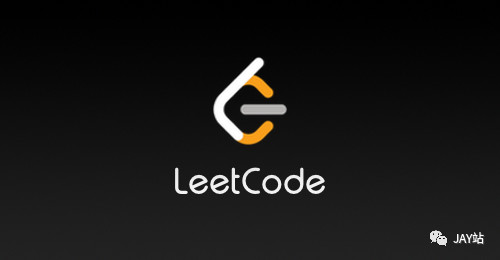February 24, 2020

# LeetCode 91 解码方法### 91. 解码方法

Difficulty: 中等

``````'A' -> 1
'B' -> 2
...
'Z' -> 26
``````

``````输入: "12"

``````

``````输入: "226"

``````

### Solution

Language: C++

#### 暴力求解之路

``````​
class Solution
{
public:
int total;
int numDecodings(string s)
{
if (s.empty() || s == '0')
return 0;
// 暴力的方式, 回溯算法 截取一个或者两个
total = 0;
numDecodings(s, 0, s.length());
}

void numDecodings(string &s, int cur, int end)
{
if (cur == end)
{
total++;
return;
}
// 选择
if (s[cur] == '0')
{
return;
}
else if (s[cur] == '1')
{
if (cur <= (end - 2))
{ // 可以选两个
numDecodings(s, cur + 1, end);
numDecodings(s, cur + 2, end);
}
else
{
numDecodings(s, cur + 1, end);
}
}
else if (s[cur] == '2')
{
if (cur <= (end - 2) && s[cur + 1] <= '6')
{ // 可以选两个
numDecodings(s, cur + 1, end);
numDecodings(s, cur + 2, end);
}
else
{
numDecodings(s, cur + 1, end);
}
}
else
{
numDecodings(s, cur + 1, end);
}
}
};
``````

`223 / 258 个通过测试用例` ,卡住的测试用例

``````"9371597631128776948387197132267188677349946742344217846154932859125134924241649584251978418763151253"
``````

``````class Solution
{
public:
int total;
int numDecodings(string s)
{
if (s.empty() || s == '0')
return 0;
total = 0;
numDecodings(s, 0, s.length());
}

void numDecodings(string &s, int cur, int end)
{
// 能走到最后,可能说明解码成功了
if (cur == end)
{
total++;
return;
}
// 无效解码
if (s[cur] == '0') return;
//如果可以选两个进行解码
if (cur <= (end - 2)) {
if (s[cur] == '1' ||
(s[cur] == '2' && s[cur + 1] <= '6')) {
numDecodings(s, cur + 2, end);
}
}
// 绝对可以解码1个的
numDecodings(s, cur + 1, end);
}
};

``````

``````258 / 258 个通过测试用例

``````

#### 合理的递归 与 记忆优化

``````class Solution
{
public:
int numDecodings(string s)
{
if (s.empty() || s == '0')
return 0;
if (s.length() == 1)
return 1;
return numDecodings(s, 0, s.length() - 1);
}

int numDecodings(string &s, int begin, int end)
{
// 无效解码
if (s[begin] == '0')
return 0;
// 仅一位数字 (排除了'0'),有效解码
if (end - begin <= 0)
return 1;
// 起码两位长度的字符串了
// 先取一位
int count = numDecodings(s, begin + 1, end);
const int value = stoi(s.substr(begin, 2));
// 再取两位
if (value <= 26)
count += numDecodings(s, begin + 2, end);
return count;
}
};

239 / 258 个通过测试用例 卡在了
"1787897759966261825913315262377298132516969578441236833255596967132573482281598412163216914566534565"

``````

``````class Solution
{
public:
int numDecodings(string s)
{
if (s.empty() || s == '0')
return 0;
if (s.length() == 1)
return 1;
return numDecodings(s, 0, s.length() - 1);
}

int numDecodings(string &s, int begin, int end)
{
// 无效解码
if (s[begin] == '0')
return 0;
// 仅一位数字 (排除了'0'),有效解码
if (end - begin <= 0)
return 1;
// 起码两位长度的字符串了
// 先取一位
int count = numDecodings(s, begin + 1, end);
// stoi时间复杂度太高, 导致提交失败ಥ_ಥ
// 再取两位
if (s[begin] == '1'
|| (s[begin] == '2' && s[begin+1] <= '6'))
count += numDecodings(s, begin + 2, end);
return count;
}
};

258 / 258 个通过测试用例

``````

``````class Solution
{
public:
// 比使用数组效果更好
unordered_map<int,int> memo;
int numDecodings(string s)
{
if (s.empty())
return 0;
return numDecodings(s, 0, s.length() - 1);
}

private:
int numDecodings(const string &s, int begin, int end)
{
if (memo.count(begin))
return memo[begin];
// 无效解码
if (s[begin] == '0')
return 0;
// 仅一位数字 (排除了'0'),有效解码
if (begin>=end)
return 1;
// 起码两位长度的字符串了
// 先取一位
int count = numDecodings(s, begin + 1, end);
// 再取两位 查看花花酱大神的代码实现,发现简单实现 stoi 效率比直接判断字符还高 ಥ_ಥ
const int prefix = (s[begin] - '0') * 10 + (s[begin + 1] - '0');
if (prefix <= 26)
count += numDecodings(s, begin + 2, end);
// 记忆
memo[begin] = count;
return count;
}
};

``````

#### 动态规划

• 找状态定义, 不同的状态定义可能导致不同的时间复杂度;
• 状态定义要包含 变化的条件
• 这里定义为: dp[i] 为 索引 0~i的子字符串的解码数量
• 找状态迁移方程
• 当前索引 i 的字符串 可以从 i-1的位置解码过来, 或者 i-2的解码位置过来
• 也就是 dp[i] = dp[i-1] + dp[i-2] 的值

``````class Solution {
public:
int numDecodings(string s)
{
if (s.empty() || s == '0')
return 0;
if (s.length() == 1) return 1;
// 定义 dp[i] 为从0~i的子字符串的解码情况
vector<int> dp(s.length(),0);
// 空字符串 解码为一种
// dp[-1] = 1; 不支持 索引为-1,所以后面要注意dp[i-2]的判断
// 单个字符的话(排除了'0') 解码为一种
dp = 1;
/// 我们仅仅需要累计 解码一个字符与解码两个字符 的情况即可
for (int i = 1; i< s.length();++i) {
if (!isValid(s[i]) && !isValid(s[i-1],s[i])) { // 如果s[i] 无效 且 s[i]s[i-1] 那么不管s[i-2]如何大,都没用,后面的两个数值都无法完成解码
// dp[i] = 0 + 0; // 这里直接就可返回了,很明显当前无解,后面也无解
return 0;
} else if (!isValid(s[i]) && isValid(s[i-1],s[i])) { // 如果 s[i] 无效, s[i-1][i] 有效, 说明两个字符的解码成功, 一个字符的解码失败
dp[i] = 0 + (i == 1 ? 1 : dp[i-2]);
} else if (isValid(s[i]) && !isValid(s[i-1],s[i])) { // 如果 s[i] 有效 s[i-1]s[i] 无效 ,那么 说明两个字符的没有解码成功, 一个字符的解码成功了
dp[i] = dp[i-1] + 0;
} else { // 肯定是 两个都有效了
dp[i] = dp[i-1]  + (i == 1 ? 1 : dp[i-2]);
}
}
return dp[s.length() - 1];
};
private:
bool isValid(const char c) { return c != '0'; }
bool isValid(const char c1, const char c2) {
const int num = (c1 - '0')*10 + (c2 - '0');
return num >= 10 && num <= 26;
}
};

``````

``````class Solution {
public:
int numDecodings(string s)
{
if (s.empty() || s == '0')
return 0;
if (s.length() == 1) return 1;
// 空字符串 解码为一种
int pre0 = 1; // dp[-1]
int pre1 = 1; // dp
for (int i = 1; i< s.length();++i) {
int temp = 0; // 当前的解码值
if (!isValid(s[i]) && !isValid(s[i-1],s[i])) {
return 0;
} else if (!isValid(s[i]) && isValid(s[i-1],s[i])) {
temp = pre0;
} else if (isValid(s[i]) && !isValid(s[i-1],s[i])) {
temp = pre1;
} else {
temp = pre0 + pre1;
}
// 更新
pre0 = pre1;
// 最后的值
pre1 = temp;
}
return pre1;
};
private:
bool isValid(const char c) { return c != '0'; }
bool isValid(const char c1, const char c2) {
const int num = (c1 - '0')*10 + (c2 - '0');
return num >= 10 && num <= 26;
}
};

``````